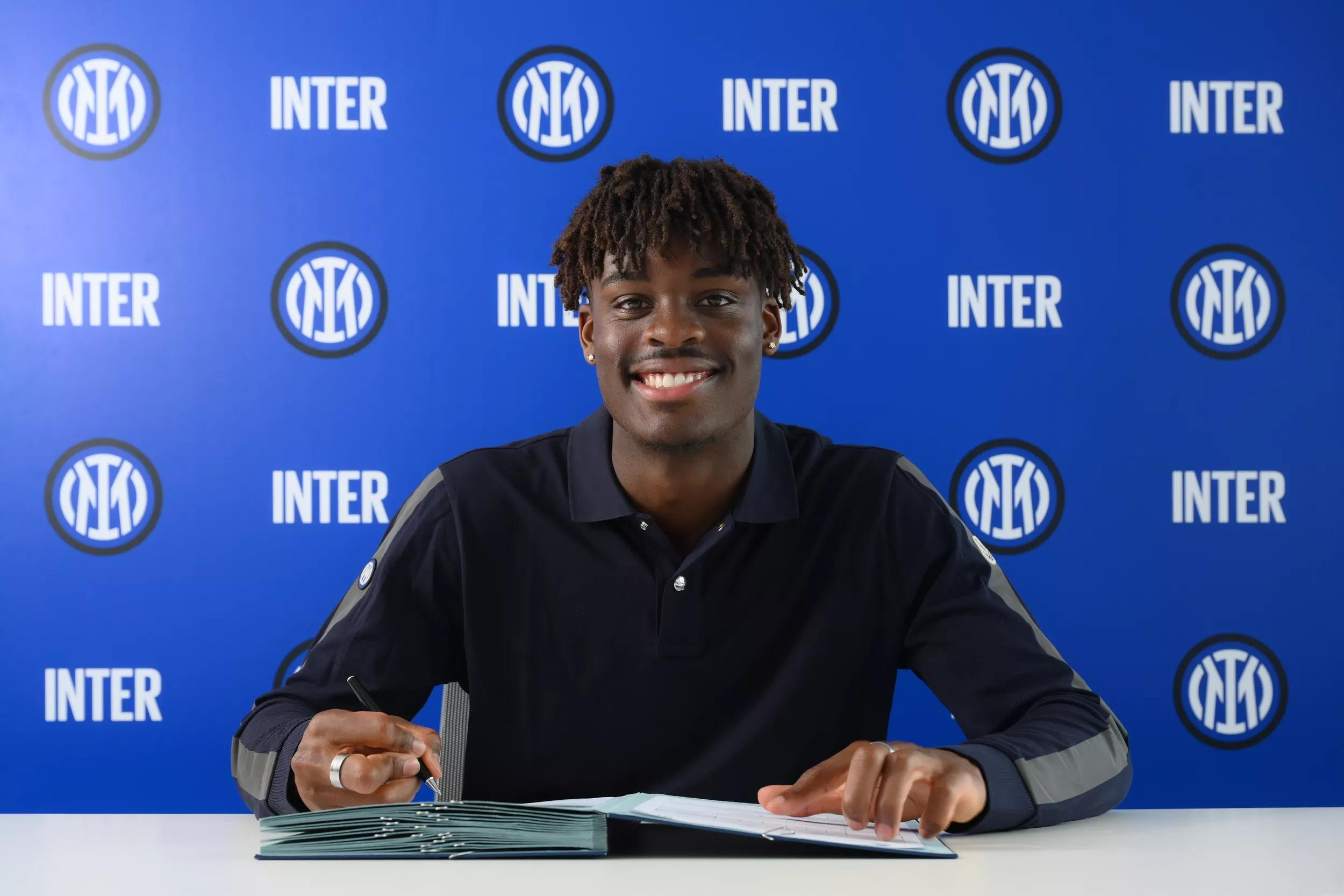# .css-adzqt3{display:inline-block;word-break:break-word;opacity:0;position:relative;-webkit-animation:animation-plwpox 0ms 0.4s forwards;animation:animation-plwpox 0ms 0.4s forwards;}.css-adzqt3::after{content:"";position:absolute;width:calc(100% + 14px);height:100%;top:0px;left:0px;background-color:var(--chakra-colors-light-white_100);-webkit-transform:scale3d(0, 1.05, 1);-moz-transform:scale3d(0, 1.05, 1);-ms-transform:scale3d(0, 1.05, 1);transform:scale3d(0, 1.05, 1);-webkit-animation:animation-f981je 250ms 0.15000000000000002s forwards step-end,animation-1ncn112 250ms 0.65s forwards step-end;animation:animation-f981je 250ms 0.15000000000000002s forwards step-end,animation-1ncn112 250ms 0.65s forwards step-end;}@-webkit-keyframes animation-1ncn112{0%{-webkit-transform:scale3d(0.75, 1.05, 1);-moz-transform:scale3d(0.75, 1.05, 1);-ms-transform:scale3d(0.75, 1.05, 1);transform:scale3d(0.75, 1.05, 1);transform-origin:top right;}44.4%{-webkit-transform:scale3d(0.25, 1.05, 1);-moz-transform:scale3d(0.25, 1.05, 1);-ms-transform:scale3d(0.25, 1.05, 1);transform:scale3d(0.25, 1.05, 1);transform-origin:top right;}100%{-webkit-transform:scale3d(0, 1.05, 1);-moz-transform:scale3d(0, 1.05, 1);-ms-transform:scale3d(0, 1.05, 1);transform:scale3d(0, 1.05, 1);transform-origin:top right;}}@keyframes animation-1ncn112{0%{-webkit-transform:scale3d(0.75, 1.05, 1);-moz-transform:scale3d(0.75, 1.05, 1);-ms-transform:scale3d(0.75, 1.05, 1);transform:scale3d(0.75, 1.05, 1);transform-origin:top right;}44.4%{-webkit-transform:scale3d(0.25, 1.05, 1);-moz-transform:scale3d(0.25, 1.05, 1);-ms-transform:scale3d(0.25, 1.05, 1);transform:scale3d(0.25, 1.05, 1);transform-origin:top right;}100%{-webkit-transform:scale3d(0, 1.05, 1);-moz-transform:scale3d(0, 1.05, 1);-ms-transform:scale3d(0, 1.05, 1);transform:scale3d(0, 1.05, 1);transform-origin:top right;}}@-webkit-keyframes animation-f981je{0%{-webkit-transform:scale3d(0, 1.05, 1);-moz-transform:scale3d(0, 1.05, 1);-ms-transform:scale3d(0, 1.05, 1);transform:scale3d(0, 1.05, 1);transform-origin:top left;}22.2%{-webkit-transform:scale3d(0.25, 1.05, 1);-moz-transform:scale3d(0.25, 1.05, 1);-ms-transform:scale3d(0.25, 1.05, 1);transform:scale3d(0.25, 1.05, 1);transform-origin:top left;}44.4%{-webkit-transform:scale3d(0.5, 1.05, 1);-moz-transform:scale3d(0.5, 1.05, 1);-ms-transform:scale3d(0.5, 1.05, 1);transform:scale3d(0.5, 1.05, 1);transform-origin:top left;}66.6%{-webkit-transform:scale3d(0.75, 1.05, 1);-moz-transform:scale3d(0.75, 1.05, 1);-ms-transform:scale3d(0.75, 1.05, 1);transform:scale3d(0.75, 1.05, 1);transform-origin:top left;}100%{-webkit-transform:scale3d(1, 1.05, 1);-moz-transform:scale3d(1, 1.05, 1);-ms-transform:scale3d(1, 1.05, 1);transform:scale3d(1, 1.05, 1);transform-origin:top left;}}@keyframes animation-f981je{0%{-webkit-transform:scale3d(0, 1.05, 1);-moz-transform:scale3d(0, 1.05, 1);-ms-transform:scale3d(0, 1.05, 1);transform:scale3d(0, 1.05, 1);transform-origin:top left;}22.2%{-webkit-transform:scale3d(0.25, 1.05, 1);-moz-transform:scale3d(0.25, 1.05, 1);-ms-transform:scale3d(0.25, 1.05, 1);transform:scale3d(0.25, 1.05, 1);transform-origin:top left;}44.4%{-webkit-transform:scale3d(0.5, 1.05, 1);-moz-transform:scale3d(0.5, 1.05, 1);-ms-transform:scale3d(0.5, 1.05, 1);transform:scale3d(0.5, 1.05, 1);transform-origin:top left;}66.6%{-webkit-transform:scale3d(0.75, 1.05, 1);-moz-transform:scale3d(0.75, 1.05, 1);-ms-transform:scale3d(0.75, 1.05, 1);transform:scale3d(0.75, 1.05, 1);transform-origin:top left;}100%{-webkit-transform:scale3d(1, 1.05, 1);-moz-transform:scale3d(1, 1.05, 1);-ms-transform:scale3d(1, 1.05, 1);transform:scale3d(1, 1.05, 1);transform-origin:top left;}}@-webkit-keyframes animation-plwpox{from{opacity:0;}to{opacity:1;}}@keyframes animation-plwpox{from{opacity:0;}to{opacity:1;}}扬.css-z4mzdx{display:inline-block;word-break:break-word;opacity:0;position:relative;-webkit-animation:animation-plwpox 0ms 0.65s forwards;animation:animation-plwpox 0ms 0.65s forwards;}.css-z4mzdx::after{content:"";position:absolute;width:calc(100% + 14px);height:100%;top:0px;left:0px;background-color:var(--chakra-colors-light-white_100);-webkit-transform:scale3d(0, 1.05, 1);-moz-transform:scale3d(0, 1.05, 1);-ms-transform:scale3d(0, 1.05, 1);transform:scale3d(0, 1.05, 1);-webkit-animation:animation-f981je 250ms 0.4s forwards step-end,animation-1ncn112 250ms 0.9s forwards step-end;animation:animation-f981je 250ms 0.4s forwards step-end,animation-1ncn112 250ms 0.9s forwards step-end;}@-webkit-keyframes animation-1ncn112{0%{-webkit-transform:scale3d(0.75, 1.05, 1);-moz-transform:scale3d(0.75, 1.05, 1);-ms-transform:scale3d(0.75, 1.05, 1);transform:scale3d(0.75, 1.05, 1);transform-origin:top right;}44.4%{-webkit-transform:scale3d(0.25, 1.05, 1);-moz-transform:scale3d(0.25, 1.05, 1);-ms-transform:scale3d(0.25, 1.05, 1);transform:scale3d(0.25, 1.05, 1);transform-origin:top right;}100%{-webkit-transform:scale3d(0, 1.05, 1);-moz-transform:scale3d(0, 1.05, 1);-ms-transform:scale3d(0, 1.05, 1);transform:scale3d(0, 1.05, 1);transform-origin:top right;}}@keyframes animation-1ncn112{0%{-webkit-transform:scale3d(0.75, 1.05, 1);-moz-transform:scale3d(0.75, 1.05, 1);-ms-transform:scale3d(0.75, 1.05, 1);transform:scale3d(0.75, 1.05, 1);transform-origin:top right;}44.4%{-webkit-transform:scale3d(0.25, 1.05, 1);-moz-transform:scale3d(0.25, 1.05, 1);-ms-transform:scale3d(0.25, 1.05, 1);transform:scale3d(0.25, 1.05, 1);transform-origin:top right;}100%{-webkit-transform:scale3d(0, 1.05, 1);-moz-transform:scale3d(0, 1.05, 1);-ms-transform:scale3d(0, 1.05, 1);transform:scale3d(0, 1.05, 1);transform-origin:top right;}}@-webkit-keyframes animation-f981je{0%{-webkit-transform:scale3d(0, 1.05, 1);-moz-transform:scale3d(0, 1.05, 1);-ms-transform:scale3d(0, 1.05, 1);transform:scale3d(0, 1.05, 1);transform-origin:top left;}22.2%{-webkit-transform:scale3d(0.25, 1.05, 1);-moz-transform:scale3d(0.25, 1.05, 1);-ms-transform:scale3d(0.25, 1.05, 1);transform:scale3d(0.25, 1.05, 1);transform-origin:top left;}44.4%{-webkit-transform:scale3d(0.5, 1.05, 1);-moz-transform:scale3d(0.5, 1.05, 1);-ms-transform:scale3d(0.5, 1.05, 1);transform:scale3d(0.5, 1.05, 1);transform-origin:top left;}66.6%{-webkit-transform:scale3d(0.75, 1.05, 1);-moz-transform:scale3d(0.75, 1.05, 1);-ms-transform:scale3d(0.75, 1.05, 1);transform:scale3d(0.75, 1.05, 1);transform-origin:top left;}100%{-webkit-transform:scale3d(1, 1.05, 1);-moz-transform:scale3d(1, 1.05, 1);-ms-transform:scale3d(1, 1.05, 1);transform:scale3d(1, 1.05, 1);transform-origin:top left;}}@keyframes animation-f981je{0%{-webkit-transform:scale3d(0, 1.05, 1);-moz-transform:scale3d(0, 1.05, 1);-ms-transform:scale3d(0, 1.05, 1);transform:scale3d(0, 1.05, 1);transform-origin:top left;}22.2%{-webkit-transform:scale3d(0.25, 1.05, 1);-moz-transform:scale3d(0.25, 1.05, 1);-ms-transform:scale3d(0.25, 1.05, 1);transform:scale3d(0.25, 1.05, 1);transform-origin:top left;}44.4%{-webkit-transform:scale3d(0.5, 1.05, 1);-moz-transform:scale3d(0.5, 1.05, 1);-ms-transform:scale3d(0.5, 1.05, 1);transform:scale3d(0.5, 1.05, 1);transform-origin:top left;}66.6%{-webkit-transform:scale3d(0.75, 1.05, 1);-moz-transform:scale3d(0.75, 1.05, 1);-ms-transform:scale3d(0.75, 1.05, 1);transform:scale3d(0.75, 1.05, 1);transform-origin:top left;}100%{-webkit-transform:scale3d(1, 1.05, 1);-moz-transform:scale3d(1, 1.05, 1);-ms-transform:scale3d(1, 1.05, 1);transform:scale3d(1, 1.05, 1);transform-origin:top left;}}@-webkit-keyframes animation-plwpox{from{opacity:0;}to{opacity:1;}}@keyframes animation-plwpox{from{opacity:0;}to{opacity:1;}}-.css-1hstmlz{display:inline-block;word-break:break-word;opacity:0;position:relative;-webkit-animation:animation-plwpox 0ms 0.9s forwards;animation:animation-plwpox 0ms 0.9s forwards;}.css-1hstmlz::after{content:"";position:absolute;width:calc(100% + 14px);height:100%;top:0px;left:0px;background-color:var(--chakra-colors-light-white_100);-webkit-transform:scale3d(0, 1.05, 1);-moz-transform:scale3d(0, 1.05, 1);-ms-transform:scale3d(0, 1.05, 1);transform:scale3d(0, 1.05, 1);-webkit-animation:animation-f981je 250ms 0.65s forwards step-end,animation-1ncn112 250ms 1.15s forwards step-end;animation:animation-f981je 250ms 0.65s forwards step-end,animation-1ncn112 250ms 1.15s forwards step-end;}@-webkit-keyframes animation-1ncn112{0%{-webkit-transform:scale3d(0.75, 1.05, 1);-moz-transform:scale3d(0.75, 1.05, 1);-ms-transform:scale3d(0.75, 1.05, 1);transform:scale3d(0.75, 1.05, 1);transform-origin:top right;}44.4%{-webkit-transform:scale3d(0.25, 1.05, 1);-moz-transform:scale3d(0.25, 1.05, 1);-ms-transform:scale3d(0.25, 1.05, 1);transform:scale3d(0.25, 1.05, 1);transform-origin:top right;}100%{-webkit-transform:scale3d(0, 1.05, 1);-moz-transform:scale3d(0, 1.05, 1);-ms-transform:scale3d(0, 1.05, 1);transform:scale3d(0, 1.05, 1);transform-origin:top right;}}@keyframes animation-1ncn112{0%{-webkit-transform:scale3d(0.75, 1.05, 1);-moz-transform:scale3d(0.75, 1.05, 1);-ms-transform:scale3d(0.75, 1.05, 1);transform:scale3d(0.75, 1.05, 1);transform-origin:top right;}44.4%{-webkit-transform:scale3d(0.25, 1.05, 1);-moz-transform:scale3d(0.25, 1.05, 1);-ms-transform:scale3d(0.25, 1.05, 1);transform:scale3d(0.25, 1.05, 1);transform-origin:top right;}100%{-webkit-transform:scale3d(0, 1.05, 1);-moz-transform:scale3d(0, 1.05, 1);-ms-transform:scale3d(0, 1.05, 1);transform:scale3d(0, 1.05, 1);transform-origin:top right;}}@-webkit-keyframes animation-f981je{0%{-webkit-transform:scale3d(0, 1.05, 1);-moz-transform:scale3d(0, 1.05, 1);-ms-transform:scale3d(0, 1.05, 1);transform:scale3d(0, 1.05, 1);transform-origin:top left;}22.2%{-webkit-transform:scale3d(0.25, 1.05, 1);-moz-transform:scale3d(0.25, 1.05, 1);-ms-transform:scale3d(0.25, 1.05, 1);transform:scale3d(0.25, 1.05, 1);transform-origin:top left;}44.4%{-webkit-transform:scale3d(0.5, 1.05, 1);-moz-transform:scale3d(0.5, 1.05, 1);-ms-transform:scale3d(0.5, 1.05, 1);transform:scale3d(0.5, 1.05, 1);transform-origin:top left;}66.6%{-webkit-transform:scale3d(0.75, 1.05, 1);-moz-transform:scale3d(0.75, 1.05, 1);-ms-transform:scale3d(0.75, 1.05, 1);transform:scale3d(0.75, 1.05, 1);transform-origin:top left;}100%{-webkit-transform:scale3d(1, 1.05, 1);-moz-transform:scale3d(1, 1.05, 1);-ms-transform:scale3d(1, 1.05, 1);transform:scale3d(1, 1.05, 1);transform-origin:top left;}}@keyframes animation-f981je{0%{-webkit-transform:scale3d(0, 1.05, 1);-moz-transform:scale3d(0, 1.05, 1);-ms-transform:scale3d(0, 1.05, 1);transform:scale3d(0, 1.05, 1);transform-origin:top left;}22.2%{-webkit-transform:scale3d(0.25, 1.05, 1);-moz-transform:scale3d(0.25, 1.05, 1);-ms-transform:scale3d(0.25, 1.05, 1);transform:scale3d(0.25, 1.05, 1);transform-origin:top left;}44.4%{-webkit-transform:scale3d(0.5, 1.05, 1);-moz-transform:scale3d(0.5, 1.05, 1);-ms-transform:scale3d(0.5, 1.05, 1);transform:scale3d(0.5, 1.05, 1);transform-origin:top left;}66.6%{-webkit-transform:scale3d(0.75, 1.05, 1);-moz-transform:scale3d(0.75, 1.05, 1);-ms-transform:scale3d(0.75, 1.05, 1);transform:scale3d(0.75, 1.05, 1);transform-origin:top left;}100%{-webkit-transform:scale3d(1, 1.05, 1);-moz-transform:scale3d(1, 1.05, 1);-ms-transform:scale3d(1, 1.05, 1);transform:scale3d(1, 1.05, 1);transform-origin:top left;}}@-webkit-keyframes animation-plwpox{from{opacity:0;}to{opacity:1;}}@keyframes animation-plwpox{from{opacity:0;}to{opacity:1;}}比塞克加盟国际米兰

team

Jul 12th 2023

## 比塞克加盟国际米兰，签约至2028年。

2000年11月29日，扬在科隆出生，他的父母是喀麦隆人，从小他的梦想是成为一名医生，足球相对来说只是乐趣：直至在科隆U17，比塞克开始真正相信自己的能力，这要归功于马库斯-道恩教练的信任。正是在这里，扬获得了突飞猛进的成长，他在2017年对阵柏林赫塔的比赛中完成了自己的一线队首秀，年仅16岁零11个月，成为德甲历史上最年轻的出场球员。在90分钟的比赛里，扬的传球成功率达到96%，并赢得了所有对抗，尤其是在头球上压制了塞尔克（德国国脚，高中锋），充分证明了他的能力。

NEWS

Let's get to know Yann-Aurel Bisseck## 相关新闻team

Yesterday

• 俱乐部

• Inter Store